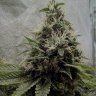# How To Calculate Ppm From Fertilizers

• None#### xinobyteAbove is an example of a nutrient labels guaranteed analysis.
It tells us how much of each element is in the bag at percentage weight by volume (%w/v).
This provides us with enough information to establish a reasonably accurate ppm.

Note that analyzing the ppm from fertilizer labels won't provide 100% accurate ppms.
Fertilizers sold worldwide are often only required to be listed accurately to within 0.4%.

Regulations around the world require that NPK.. values be presented somewhat ambiguously.
Therefore, listings for the same nutrient may appear to vary on a country-by-country basis.
For example, when looking at our labels guaranteed analysis you will find note that it states;

Available Phosphate (P2O5)..........15.0%
Available Potash (K2O)..............14.0%

This information becomes important when interpreting the guaranteed analysis.

That is, it is important to note that the P and K numbers found on the guaranteed analysis do not always reflect the actual amounts of elemental phosphorous and potassium by %.

With our label, this is the case and P is listed as P2O5 (phosphorous pentoxide) and K is listed as K2O (potassium oxide) percentage.

When phosphorus is listed as P2O5 it is only 43% elemental P and when potassium is listed as K2O it is only 83% elemental K.

Therefore, when this system is in use, a 5-15-14 NPK ratio truly reflects elemental NPK 5-6.45-11.62.

N = 5
P = 15 * 0.43 = 6.45
K = 14 * 0.83 = 11.62

Additionally, other nutrients such as calcium (Ca), magnesium (Mg) and sulfur (S) can be listed in their oxide form (CaO, MgO, SO3) or in elemental form, or both.
To convert other nutrient listings that may appear on some labels use these equations.

CaO to Ca multiply by 0.714
MgO to Mg multiply by 0.6031
SO3 to S multiply by 0.4

Percentage Weight by Volume (%w/v)

A simple way of understanding how to convert a %w/v listing found on the guaranteed analysis into grams per litre is by understanding that 1ml of RO water weighs 1gram.

Percentage weight by volume %w/v refers to the total weight of elements contained within a finished concentrate of a given total volume.

For example, 5% of nitrogen added to 1 litre(1000ml) of RO water would mean that there is 50grams of N in the water.

1000 (ml) * 0.05 (5% nitrogen) = 50 (grams of N)

Converting %w/v to ppm and ppm to %w/v

To establish ppm from %w/v you simply need to multiply by 10000.
5% (nitrogen) * 10000 = 50000 (ppm)
To establish %w/v from ppm you simply need to divide by 10000.
50000 (ppm) / 10000 = 5% (nitrogen)

To establish the concentration of individual elements in the water, the guaranteed analysis (%w/v) should first be converted into ppm, then multiplied by the usage rate (per litre), then divided by 1000 (ml).

For example, if a nutrient lists 5% nitrogen, when it is used at 5grams per 4 litres it will yield 62.5 ppm of nitrogen per litre.

Step 1 : 5% (nitrogen) * 10000 = 50000 (ppm)
Step 2 : 5grams / 4L = 1.25g/litre
Step 3 : (50000 (ppm) * 1.25g/litre) / 1000ml (1 litre) = 62.5ppm of nitrogen per litre (1000ml)

Doing the math

Using what we've learned, we're finally ready to find the ppm of our fertilizer.

5 * 10000 = 50k nitrogen ppm
6.45 * 10000 = 64.5k phosphorus ppm
11.62 * 10000 = 116.2k potassium
5 * 10000 = 50k calcium ppm
3.5 * 10000 = 35k magnesium ppm
4 * 10000 = 40k sulfur ppm
0.1 * 10000 = 1k iron ppm

50k + 64.5k + 116.2k + 50k + 35k + 40k + 1k = 356,7k or 356700 ppm.
(356700ppm * 1.25g/litre) / 1000ml = 445ppm(~0.9EC) per litre.#### JWM2

Supporter
Great post! Very informative and useful. Kudos.#### 1diesel1

Staff
Supporter

•Smoking Gun#### xinobyte

You can also calculate your desired ppm this way using basic arithmetic.
I use this all the time, it's extremely useful.

Xinobyte's tap water is 150ppm and wants to water his plants at 400ppm.
How many grams of fertilizer per litre should he uses?
Note that his pH down adds up 20ppm.

Equation

400ppm - 150ppm - 20ppm = 230ppm
230000ppm / 356700ppm = 0.64g/litre

Proof

(356700ppm * 0.64g/litre) / 1000ml = 228ppm per litre.
Xinobyte should use 0.64g per litre.#### Erick31876

Thanks for sharing this. Very useful

•Smoking Gun#### cemchris

Supporter
•Smoking Gun and xinobyte